﻿ 具有输入饱和的严格反馈系统的约束控制 Constrained Control for Strict Feedback Systems with Input Saturation

Modeling and Simulation
Vol. 08  No. 03 ( 2019 ), Article ID: 31887 , 15 pages
10.12677/MOS.2019.83013

Constrained Control for Strict Feedback Systems with Input Saturation

Li Tang, Jiyang Jia

College of Science, Liaoning University of Technology, Jinzhou LiaoningReceived: Aug. 2nd, 2019; accepted: Aug. 19th, 2019; published: Aug. 26th, 2019ABSTRACT

An adaptive neural network tracking control scheme is proposed for nonlinear strict feedback systems with full state constraints and input saturation. The adaptive controller is designed by combining the backstepping technique and neural network approximator. In the design process, firstly, a continuous differentiable asymmetric saturation model is constructed by using Gaussian error function. Secondly, tangent Barrier Lyapunov functions (tan-BLFs) are selected to deal with the state constraint problems, that is to ensure that the full-state constraint is not violated. Compared with existing logarithm-BLFs and integral-BLFs, tan-BLFs are the BLFs that can deal with both constrained and unconstrained systems. Thirdly, it is proved by the theorem that all the signals in the closed-loop systems are uniformly ultimately bounded, and the error signals are kept in the small neighborhood of zero, and full state constraints are not violated. Finally, the simulation results show that the proposed scheme is effective and feasible.

Keywords:Adaptive Control, Full State Constraints, Barrier Lyapunov Functions

Email: tangli0413@163.com1. 引言

2. 问题描述及稳定性分析

2.1. 系统描述

$\left\{\begin{array}{l}\begin{array}{cc}{\stackrel{˙}{x}}_{i}={f}_{i}\left({\stackrel{¯}{x}}_{i}\right)+{g}_{i}\left({\stackrel{¯}{x}}_{i}\right){x}_{i+1}& i=1,\cdots ,n-1\end{array}\\ {\stackrel{˙}{x}}_{n}={f}_{n}\left({\stackrel{¯}{x}}_{n}\right)+{g}_{n}\left({\stackrel{¯}{x}}_{n}\right)u\left(v\right)\\ y={x}_{1}\end{array}$ (1)

$u\left(v\right)={u}_{M}×erf\left(\frac{\sqrt{\text{π}}}{2{u}_{M}}v\right)$ (2)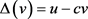$u=cv+\Delta \left(v\right)$ (3)(4)

2.2. 控制器设计和系统稳定性分析

$\begin{array}{c}{\stackrel{˙}{z}}_{1}={\stackrel{˙}{x}}_{1}-{\stackrel{˙}{y}}_{d}\\ ={f}_{1}\left({x}_{1}\right)+{g}_{1}\left({x}_{1}\right){x}_{2}-{\stackrel{˙}{y}}_{d}\\ ={f}_{1}\left({x}_{1}\right)+{g}_{1}\left({x}_{1}\right)\left({z}_{2}+{\alpha }_{1}\right)-{\stackrel{˙}{y}}_{d}\end{array}$ (5)

${V}_{1}$ 对时间t求导，则得到 ${\stackrel{˙}{V}}_{1}$ 的表示如下

${\stackrel{˙}{V}}_{1}=z\left({f}_{1}\left({x}_{1}\right)+{g}_{1}\left({x}_{1}\right)\left({z}_{2}+{\alpha }_{1}\right)-{\stackrel{˙}{y}}_{d}\right){\mathrm{sec}}^{2}\left(\frac{\text{π}{z}_{1}^{2}}{2{k}_{{b}_{1}}^{2}}\right)$ (6)

${f}_{1}\left({x}_{1}\right)-{\stackrel{˙}{y}}_{d}={W}_{1}^{\text{T}}{S}_{1}\left({Z}_{1}\right)+{\epsilon }_{1}\left({Z}_{1}\right)$ (7)

${\stackrel{˙}{V}}_{1}={z}_{1}\left({g}_{1}\left({x}_{1}\right){z}_{2}+{W}_{1}^{\text{T}}{S}_{1}\left({Z}_{1}\right)+{\epsilon }_{1}\left({Z}_{1}\right)\right){\mathrm{sec}}^{2}\left(\frac{\text{π}{z}_{1}^{2}}{2{k}_{{b}_{1}}^{2}}\right)$ (8)

$\left\{\begin{array}{l}{z}_{1}{W}_{1}^{\text{T}}{S}_{1}\left({Z}_{1}\right){\mathrm{sec}}^{2}\left(\frac{\text{π}{z}_{1}^{2}}{2{k}_{{b}_{1}}^{2}}\right)\le \frac{1}{2}{a}_{1}^{2}+\frac{{\Theta }_{1}}{2{a}_{1}^{2}}{S}_{1}^{\text{T}}\left({Z}_{1}\right){S}_{1}\left({Z}_{1}\right){z}_{1}^{2}{\mathrm{sec}}^{4}\left(\frac{\text{π}{z}_{1}^{2}}{2{k}_{{b}_{1}}^{2}}\right)\\ {z}_{1}{\epsilon }_{1}\left({Z}_{1}\right){\mathrm{sec}}^{2}\left(\frac{\text{π}{z}_{1}^{2}}{2{k}_{{b}_{1}}^{2}}\right)\le \frac{1}{2}{\epsilon }_{1}^{*2}+\frac{1}{2}{z}_{1}^{2}{\mathrm{sec}}^{4}\left(\frac{\text{π}{z}_{1}^{2}}{2{k}_{{b}_{1}}^{2}}\right)\\ {g}_{1}\left({x}_{1}\right){z}_{1}{z}_{2}{\mathrm{sec}}^{2}\left(\frac{\text{π}{z}_{1}^{2}}{2{k}_{{b}_{1}}^{2}}\right)\le \frac{1}{2}{z}_{2}^{2}+\frac{1}{2}{g}_{1}^{2}\left({x}_{1}\right){z}_{1}^{2}{\mathrm{sec}}^{4}\left(\frac{\text{π}{z}_{1}^{2}}{2{k}_{{b}_{1}}^{2}}\right)\end{array}$ (9)

${‖{W}_{\mathrm{max},1}‖}^{2}\ge {‖{W}_{1}‖}^{2}$ ，记 ${‖{W}_{\mathrm{max},1}‖}^{2}={\Theta }_{1}$ ，其中 ${\stackrel{˜}{\Theta }}_{1}={\Theta }_{1}-{\stackrel{^}{\Theta }}_{1}$ ，且 ${\stackrel{^}{\Theta }}_{1}$${\Theta }_{1}$ 的估计，将(9)代入(8)有

$\begin{array}{c}{\stackrel{˙}{V}}_{1}\le \frac{1}{2}{z}_{2}^{2}+\frac{1}{2}{g}_{1}^{2}\left({x}_{1}\right){z}_{1}^{2}{\mathrm{sec}}^{4}\left(\frac{\text{π}{z}_{1}^{2}}{2{k}_{{b}_{1}}^{2}}\right)+\frac{1}{2}{a}_{1}^{2}+\frac{1}{2}{\epsilon }_{1}^{*2}\\ \text{\hspace{0.17em}}\text{\hspace{0.17em}}+\frac{{\Theta }_{1}}{2{a}_{1}^{2}}{S}_{1}^{\text{T}}\left({Z}_{1}\right){S}_{1}\left({Z}_{1}\right){z}_{1}^{2}{\mathrm{sec}}^{4}\left(\frac{\text{π}{z}_{1}^{2}}{2{k}_{{b}_{1}}^{2}}\right)\\ \text{\hspace{0.17em}}\text{\hspace{0.17em}}+\frac{1}{2}{z}_{1}^{2}{\mathrm{sec}}^{4}\left(\frac{\text{π}{z}_{1}^{2}}{2{k}_{{b}_{1}}^{2}}\right)+{g}_{1}\left({x}_{1}\right){z}_{1}{\alpha }_{1}{\mathrm{sec}}^{2}\left(\frac{\text{π}{z}_{1}^{2}}{2{k}_{{b}_{1}}^{2}}\right)\end{array}$ (10)

$\begin{array}{l}{\alpha }_{1}=\frac{1}{{g}_{1}\left({x}_{1}\right)}\left(-\frac{{k}_{{b}_{1}}^{2}{\varphi }_{1}}{\text{π}{z}_{1}}\mathrm{cos}\left(\frac{\text{π}{z}_{1}^{2}}{2{k}_{{b}_{1}}^{2}}\right)\mathrm{sin}\left(\frac{\text{π}{z}_{1}^{2}}{2{k}_{{b}_{1}}^{2}}\right)-\frac{1}{2}{z}_{1}{\mathrm{sec}}^{2}\left(\frac{\text{π}{z}_{1}^{2}}{2{k}_{{b}_{1}}^{2}}\right)\\ \text{ }\text{ }\text{ }\text{ }\text{ }\text{ }\text{ }\text{ }\text{ }\text{ }\text{ }\text{ }\text{ }\text{ }\text{ }\text{ }\text{ }\text{ }\text{ }\text{ }-\frac{1}{2}{g}_{1}^{2}\left({x}_{1}\right){z}_{1}{\mathrm{sec}}^{2}\left(\frac{\text{π}{z}_{1}^{2}}{2{k}_{{b}_{1}}^{2}}\right)-\frac{{\stackrel{^}{\Theta }}_{1}}{2{a}_{1}^{2}}{S}_{1}^{\text{T}}\left({Z}_{1}\right){S}_{1}\left({Z}_{1}\right){z}_{1}{\mathrm{sec}}^{2}\left(\frac{\text{π}{z}_{1}^{2}}{2{k}_{{b}_{1}}^{2}}\right)\right)\end{array}$ (11)

$\begin{array}{l}{\stackrel{˙}{V}}_{1}\le \frac{1}{2}{z}_{2}^{2}+\frac{{\stackrel{˜}{\Theta }}_{1}}{2{a}_{1}^{2}}{S}_{1}^{\text{T}}\left({Z}_{1}\right){S}_{1}\left({Z}_{1}\right){z}_{1}^{2}{\mathrm{sec}}^{4}\left(\frac{\text{π}{z}_{1}^{2}}{2{k}_{{b}_{1}}^{2}}\right)\\ \text{\hspace{0.17em}}\text{\hspace{0.17em}}\text{\hspace{0.17em}}\text{\hspace{0.17em}}\text{\hspace{0.17em}}\text{\hspace{0.17em}}+\frac{1}{2}{a}_{1}^{2}+\frac{1}{2}{\epsilon }_{1}^{*2}-\frac{{k}_{b1}^{2}}{\text{π}}{\varphi }_{1}\mathrm{tan}\left(\frac{\text{π}{z}_{1}^{2}}{2{k}_{{b}_{1}}^{2}}\right)\end{array}$ (12)

$\begin{array}{c}{\stackrel{˙}{z}}_{2}={\stackrel{˙}{x}}_{2}-{\stackrel{˙}{\alpha }}_{1}\\ ={f}_{2}\left({\stackrel{¯}{x}}_{2}\right)+{g}_{2}\left({\stackrel{¯}{x}}_{2}\right){x}_{3}-{\stackrel{˙}{\alpha }}_{1}\\ ={f}_{2}\left({\stackrel{¯}{x}}_{2}\right)+{g}_{2}\left({\stackrel{¯}{x}}_{2}\right){x}_{3}-{\stackrel{˙}{\alpha }}_{1}\end{array}$ (13)

$\begin{array}{c}{\stackrel{˙}{V}}_{2}={\stackrel{˙}{V}}_{1}+{z}_{2}{\stackrel{˙}{z}}_{2}{\mathrm{sec}}^{2}\left(\frac{\text{π}{z}_{2}^{2}}{2{k}_{{b}_{2}}^{2}}\right)\\ ={z}_{2}\left({f}_{2}\left({\stackrel{¯}{x}}_{2}\right)+{g}_{2}\left({\stackrel{¯}{x}}_{2}\right)\left({z}_{3}+{\alpha }_{2}\right)-{\stackrel{˙}{\alpha }}_{1}\right){\mathrm{sec}}^{2}\left(\frac{\text{π}{z}_{2}^{2}}{2{k}_{{b}_{2}}^{2}}\right)+{\stackrel{˙}{V}}_{1}\end{array}$ (14)

${f}_{2}\left({\stackrel{¯}{x}}_{2}\right)-{\stackrel{˙}{\alpha }}_{1}={W}_{2}^{\text{T}}{S}_{2}\left({Z}_{2}\right)+{\epsilon }_{2}\left({Z}_{2}\right)$ (15)

${\stackrel{˙}{V}}_{2}={\stackrel{˙}{V}}_{1}+{z}_{2}\left({W}_{2}^{\text{T}}{S}_{2}\left({Z}_{2}\right)+{\epsilon }_{2}\left({Z}_{2}\right)+{g}_{2}\left({\stackrel{¯}{x}}_{2}\right){z}_{3}+{g}_{2}\left({\stackrel{¯}{x}}_{2}\right){\alpha }_{2}\right){\mathrm{sec}}^{2}\left(\frac{\text{π}{z}_{2}^{2}}{2{k}_{{b}_{2}}^{2}}\right)$ (16)(17)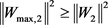，记 ${‖{W}_{\mathrm{max},2}‖}^{2}={\Theta }_{2}$ ，其中 ${\stackrel{˜}{\Theta }}_{2}={\Theta }_{2}-{\stackrel{^}{\Theta }}_{2}$ ，且 ${\stackrel{^}{\Theta }}_{2}$${\Theta }_{2}$ 的估计(17)代入(16)得

$\begin{array}{c}{\stackrel{˙}{V}}_{2}\le \frac{1}{2}{z}_{3}^{2}+\frac{1}{2}{g}_{2}^{2}\left({\stackrel{¯}{x}}_{2}\right){z}_{2}^{2}{\mathrm{sec}}^{4}\left(\frac{\text{π}{z}_{2}^{2}}{2{k}_{{b}_{2}}^{2}}\right)+\frac{1}{2}{a}_{2}^{2}+\frac{{\Theta }_{2}}{2{a}_{2}^{2}}{S}_{2}^{\text{T}}\left({Z}_{2}\right){S}_{2}\left({Z}_{2}\right){z}_{2}^{2}{\mathrm{sec}}^{4}\left(\frac{\text{π}{z}_{2}^{2}}{2{k}_{{b}_{2}}^{2}}\right)\\ \text{\hspace{0.17em}}\text{\hspace{0.17em}}+\frac{1}{2}{\epsilon }_{2}^{*2}+\frac{1}{2}{z}_{2}^{2}{\mathrm{sec}}^{4}\left(\frac{\text{π}{z}_{2}^{2}}{2{k}_{{b}_{2}}^{2}}\right)+{g}_{2}\left({\stackrel{¯}{x}}_{2}\right){\alpha }_{2}{z}_{2}{\mathrm{sec}}^{2}\left(\frac{\text{π}{z}_{2}^{2}}{2{k}_{{b}_{2}}^{2}}\right)+\frac{1}{2}{z}_{2}^{2}+\frac{1}{2}{a}_{1}^{2}\\ \text{\hspace{0.17em}}\text{\hspace{0.17em}}+\frac{{\stackrel{˜}{\Theta }}_{1}}{2{a}_{1}^{2}}{S}_{1}^{\text{T}}\left({Z}_{1}\right){S}_{1}\left({Z}_{1}\right){z}_{1}^{2}{\mathrm{sec}}^{4}\left(\frac{\text{π}{z}_{2}^{2}}{2{k}_{{b}_{2}}^{2}}\right)+\frac{1}{2}{\epsilon }_{2}^{*2}-\frac{{k}_{b1}^{2}}{\text{π}}{\varphi }_{1}\mathrm{tan}\left(\frac{\text{π}{z}_{1}^{2}}{2{k}_{{b}_{1}}^{2}}\right)\end{array}$ (18)

$\begin{array}{c}{\alpha }_{2}=\frac{1}{{g}_{2}\left({\stackrel{¯}{x}}_{2}\right)}\left(-\frac{{k}_{b2}^{2}{\varphi }_{2}}{\text{π}{z}_{2}}\mathrm{sin}\left(\frac{\text{π}{z}_{2}^{2}}{2{k}_{{b}_{2}}^{2}}\right)\mathrm{cos}\left(\frac{\text{π}{z}_{2}^{2}}{2{k}_{{b}_{2}}^{2}}\right)-\frac{{\stackrel{^}{\Theta }}_{2}}{2{a}_{2}^{2}}{S}_{2}^{\text{T}}\left({Z}_{2}\right){S}_{2}\left({Z}_{2}\right){z}_{2}{\mathrm{sec}}^{2}\left(\frac{\text{π}{z}_{2}^{2}}{2{k}_{{b}_{2}}^{2}}\right)\\ \text{\hspace{0.17em}}\text{\hspace{0.17em}}-\frac{1}{2}{z}_{2}{\mathrm{sec}}^{2}\left(\frac{\text{π}{z}_{2}^{2}}{2{k}_{{b}_{2}}^{2}}\right)-\frac{1}{2}{g}_{2}^{2}\left({\stackrel{¯}{x}}_{2}\right){z}_{2}{\mathrm{sec}}^{2}\left(\frac{\text{π}{z}_{2}^{2}}{2{k}_{{b}_{2}}^{2}}\right)-\frac{1}{2}{\text{z}}_{2}{\mathrm{cos}}^{2}\left(\frac{\text{π}{z}_{2}^{2}}{2{k}_{{b}_{2}}^{2}}\right)\right)\end{array}$ (19)

$\begin{array}{c}{\stackrel{˙}{V}}_{2}\le \underset{j=1}{\overset{2}{\sum }}\left(\frac{1}{2}{a}_{j}^{2}+\frac{1}{2}{\epsilon }_{j}^{*2}-\frac{{k}_{{b}_{j}}^{2}}{\pi }{\varphi }_{j}\mathrm{tan}\left(\frac{\text{π}{z}_{j}^{2}}{2{k}_{{b}_{j}}^{2}}\right)\\ \text{\hspace{0.17em}}\text{\hspace{0.17em}}+\frac{{\stackrel{˜}{\Theta }}_{j}}{2{a}_{j}^{2}}{S}_{j}^{\text{T}}\left({Z}_{j}\right){S}_{j}\left({Z}_{j}\right){z}_{j}^{2}{\mathrm{sec}}^{4}\left(\frac{\text{π}{z}_{j}^{2}}{2{k}_{{b}_{j}}^{2}}\right)\right)+\frac{1}{2}{z}_{3}^{2}\end{array}$ (20)

$\begin{array}{c}{\stackrel{˙}{z}}_{i}={\stackrel{˙}{x}}_{i}-{\stackrel{˙}{\alpha }}_{i-1}\\ ={f}_{i}\left({\stackrel{¯}{x}}_{i}\right)+{g}_{i}\left({\stackrel{¯}{x}}_{i}\right){x}_{i+1}-{\stackrel{˙}{\alpha }}_{i-1}\\ ={f}_{i}\left({\stackrel{¯}{x}}_{i}\right)+{g}_{i}\left({\stackrel{¯}{x}}_{i}\right)\left({z}_{i+1}+{\alpha }_{i}\right)-{\stackrel{˙}{\alpha }}_{i-1}\end{array}$ (21)

${\stackrel{˙}{\alpha }}_{i-1}=\underset{j=1}{\overset{i-1}{\sum }}\frac{\partial {\alpha }_{i-1}}{\partial {x}_{j}}{\stackrel{˙}{x}}_{j}+\underset{j=0}{\overset{i-1}{\sum }}\frac{\partial {\alpha }_{i-1}}{\partial {y}_{d}^{\left(j\right)}}{y}_{d}^{\left(j+1\right)}+\underset{j=1}{\overset{i-1}{\sum }}\frac{\partial {\alpha }_{i-1}}{\partial {\stackrel{^}{\Theta }}_{j}}{\stackrel{˙}{\stackrel{^}{\Theta }}}_{j}$

$\begin{array}{c}{\stackrel{˙}{V}}_{i}={\stackrel{˙}{V}}_{i-1}+{Z}_{i}{\stackrel{˙}{Z}}_{i}{\mathrm{sec}}^{2}\left(\frac{\text{π}{z}_{i}^{2}}{2{k}_{{b}_{i}}^{2}}\right)\\ ={\stackrel{˙}{V}}_{i-1}+{z}_{i}\left({f}_{i}\left({\stackrel{¯}{x}}_{i}\right)+{g}_{i}\left({\stackrel{¯}{x}}_{i}\right)\left({z}_{i+1}+{\alpha }_{i}\right)-{\stackrel{˙}{\alpha }}_{i-1}\right)\end{array}$ (22)

${f}_{i}\left({\stackrel{¯}{x}}_{i}\right)-{\stackrel{˙}{\alpha }}_{i-1}={W}_{i}^{\text{T}}{S}_{i}\left({Z}_{i}\right)+{\epsilon }_{i}\left({Z}_{i}\right)$ (23)

${\stackrel{˙}{V}}_{i}={\stackrel{˙}{V}}_{i-1}+{z}_{i}\left({W}_{i}^{\text{T}}{S}_{i}\left({Z}_{i}\right)+{\epsilon }_{i}\left({Z}_{i}\right)+{g}_{i}\left({\stackrel{¯}{x}}_{i}\right){z}_{i+1}+{g}_{i}\left({\stackrel{¯}{x}}_{i}\right){\alpha }_{i}\right){\mathrm{sec}}^{2}\left(\frac{\text{π}{z}_{i}^{2}}{2{k}_{{b}_{i}}^{2}}\right)$ (24)

$\left\{\begin{array}{l}{z}_{i}{W}_{i}^{\text{T}}{S}_{i}\left({Z}_{i}\right){\mathrm{sec}}^{2}\left(\frac{\text{π}{z}_{i}^{2}}{2{k}_{{b}_{i}}^{2}}\right)\le \frac{1}{2}{a}_{i}^{2}+\frac{{‖{W}_{i}‖}^{2}}{2{a}_{i}^{2}}{S}_{i}^{\text{T}}\left({Z}_{i}\right){S}_{i}\left({Z}_{i}\right){z}_{i}^{2}{\mathrm{sec}}^{4}\left(\frac{\text{π}{z}_{i}^{2}}{2{k}_{{b}_{i}}^{2}}\right)\\ \text{\hspace{0.17em}}\text{\hspace{0.17em}}\text{\hspace{0.17em}}\text{\hspace{0.17em}}\text{\hspace{0.17em}}\text{\hspace{0.17em}}\text{\hspace{0.17em}}\text{\hspace{0.17em}}\text{\hspace{0.17em}}\text{\hspace{0.17em}}\text{\hspace{0.17em}}\text{\hspace{0.17em}}\text{\hspace{0.17em}}\text{\hspace{0.17em}}\text{\hspace{0.17em}}\text{\hspace{0.17em}}\text{\hspace{0.17em}}\text{\hspace{0.17em}}\text{\hspace{0.17em}}\text{\hspace{0.17em}}\text{\hspace{0.17em}}\text{\hspace{0.17em}}\text{\hspace{0.17em}}\text{\hspace{0.17em}}\text{\hspace{0.17em}}\text{\hspace{0.17em}}\text{\hspace{0.17em}}\text{\hspace{0.17em}}\text{\hspace{0.17em}}\text{\hspace{0.17em}}\text{\hspace{0.17em}}\text{ }\text{ }\le \frac{1}{2}{a}_{i}^{2}+\frac{{‖{W}_{\mathrm{max},i}‖}^{2}}{2{a}_{i}^{2}}{S}_{i}^{T}\left({Z}_{i}\right){S}_{i}\left({Z}_{i}\right){z}_{i}^{2}{\mathrm{sec}}^{4}\left(\frac{\text{π}{z}_{i}^{2}}{2{k}_{{b}_{i}}^{2}}\right)\\ \text{\hspace{0.17em}}\text{\hspace{0.17em}}\text{\hspace{0.17em}}\text{\hspace{0.17em}}\text{\hspace{0.17em}}\text{\hspace{0.17em}}\text{\hspace{0.17em}}\text{\hspace{0.17em}}\text{\hspace{0.17em}}\text{\hspace{0.17em}}\text{\hspace{0.17em}}\text{\hspace{0.17em}}\text{\hspace{0.17em}}\text{\hspace{0.17em}}\text{\hspace{0.17em}}\text{\hspace{0.17em}}\text{\hspace{0.17em}}\text{\hspace{0.17em}}\text{\hspace{0.17em}}\text{\hspace{0.17em}}\text{\hspace{0.17em}}\text{\hspace{0.17em}}\text{\hspace{0.17em}}\text{\hspace{0.17em}}\text{\hspace{0.17em}}\text{\hspace{0.17em}}\text{\hspace{0.17em}}\text{\hspace{0.17em}}\text{\hspace{0.17em}}\text{\hspace{0.17em}}\text{\hspace{0.17em}}\text{ }\text{ }=\frac{1}{2}{a}_{i}^{2}+\frac{{\Theta }_{i}}{2{a}_{i}^{2}}{S}_{i}^{\text{T}}\left({Z}_{i}\right){S}_{i}\left({Z}_{i}\right){z}_{i}^{2}{\mathrm{sec}}^{4}\left(\frac{\text{π}{z}_{i}^{2}}{2{k}_{{b}_{i}}^{2}}\right)\\ {z}_{i}{\epsilon }_{i}\left({Z}_{i}\right){\mathrm{sec}}^{2}\left(\frac{\text{π}{z}_{i}^{2}}{2{k}_{{b}_{i}}^{2}}\right)\le \frac{1}{2}{\epsilon }_{i}^{*2}+\frac{1}{2}{z}_{i}^{2}{\mathrm{sec}}^{4}\left(\frac{\text{π}{z}_{i}^{2}}{2{k}_{{b}_{i}}^{2}}\right)\\ {g}_{i}\left({\stackrel{¯}{x}}_{i}\right){z}_{i}{z}_{i+1}{\mathrm{sec}}^{2}\left(\frac{\text{π}{z}_{i}^{2}}{2{k}_{{b}_{i}}^{2}}\right)\le \frac{1}{2}{z}_{i+1}^{2}+\frac{1}{2}{g}_{i}^{2}\left({\stackrel{¯}{x}}_{i}\right){z}_{i}^{2}{\mathrm{sec}}^{4}\left(\frac{\text{π}{z}_{i}^{2}}{2{k}_{{b}_{i}}^{2}}\right)\end{array}$ (25)

${‖{W}_{\mathrm{max},i}‖}^{2}\ge {‖{W}_{i}‖}^{2}$ ，记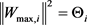，其中 ${\stackrel{˜}{\Theta }}_{i}={\Theta }_{i}-{\stackrel{^}{\Theta }}_{i}$ ，且 ${\stackrel{^}{\Theta }}_{i}$${\Theta }_{i}$ 的估计，再将(25)代入(24)得到

$\begin{array}{c}{\stackrel{˙}{V}}_{i}\le {\stackrel{˙}{V}}_{i-1}+\frac{1}{2}{z}_{i+1}^{2}+\frac{1}{2}{g}_{i}^{2}\left({\stackrel{¯}{x}}_{i}\right){z}_{i}^{2}{\mathrm{sec}}^{4}\left(\frac{\text{π}{z}_{i}^{2}}{2{k}_{{b}_{i}}^{2}}\right)\frac{1}{2}{a}_{i}^{2}\\ \text{\hspace{0.17em}}\text{\hspace{0.17em}}+\frac{{\Theta }_{i}}{2{a}_{i}^{2}}{S}_{i}^{\text{T}}\left({Z}_{i}\right){S}_{i}\left({Z}_{i}\right){z}_{i}^{2}{\mathrm{sec}}^{4}\left(\frac{\text{π}{z}_{i}^{2}}{2{k}_{{b}_{i}}^{2}}\right)+\frac{1}{2}{\epsilon }_{i}^{*2}\\ \text{\hspace{0.17em}}\text{\hspace{0.17em}}+\frac{1}{2}{z}_{i}^{2}{\mathrm{sec}}^{4}\left(\frac{\text{π}{z}_{i}^{2}}{2{k}_{{b}_{i}}^{2}}\right)+{g}_{i}\left({\stackrel{¯}{x}}_{i}\right){\alpha }_{i}{z}_{i}{\mathrm{sec}}^{2}\left(\frac{\text{π}{z}_{i}^{2}}{2{k}_{{b}_{i}}^{2}}\right)\end{array}$ (26)

$\begin{array}{c}{\alpha }_{i}=\frac{1}{{g}_{i}\left({\stackrel{¯}{x}}_{i}\right)}\left(-\frac{{k}_{bi}^{2}{\varphi }_{i}}{\text{π}{z}_{i}}\mathrm{sin}\left(\frac{\text{π}{z}_{i}^{2}}{2{k}_{bi}^{2}}\right)\mathrm{cos}\left(\frac{\text{π}{z}_{i}^{2}}{2{k}_{bi}^{2}}\right)-\frac{{\stackrel{^}{\Theta }}_{i}}{2{a}_{i}^{2}}{S}_{i}^{\text{T}}\left({Z}_{i}\right){S}_{i}\left({Z}_{i}\right){z}_{i}{\mathrm{sec}}^{2}\left(\frac{\text{π}{z}_{i}^{2}}{2{k}_{{b}_{i}}^{2}}\right)\\ \text{\hspace{0.17em}}\text{\hspace{0.17em}}-\frac{1}{2}{z}_{i}{\mathrm{sec}}^{2}\left(\frac{\text{π}{z}_{i}^{2}}{2{k}_{{b}_{i}}^{2}}\right)-\frac{1}{2}{g}_{i}^{2}\left({\stackrel{¯}{x}}_{i}\right){z}_{i}{\mathrm{sec}}^{2}\left(\frac{\text{π}{z}_{i}^{2}}{2{k}_{{b}_{i}}^{2}}\right)-\frac{1}{2}{z}_{i}{\mathrm{cos}}^{2}\left(\frac{\text{π}{z}_{i}^{2}}{2{k}_{{b}_{i}}^{2}}\right)\right)\end{array}$ (27)

$\begin{array}{c}{\stackrel{˙}{V}}_{i}\le \underset{j=1}{\overset{i}{\sum }}\left(\frac{1}{2}{a}_{j}^{2}+\frac{1}{2}{\epsilon }_{j}^{*2}-\frac{{k}_{{b}_{i}}^{2}}{\pi }{\varphi }_{j}\mathrm{tan}\left(\frac{\text{π}{z}_{j}^{2}}{2{k}_{{b}_{i}}^{2}}\right)\\ \text{\hspace{0.17em}}\text{\hspace{0.17em}}+\frac{{\stackrel{˜}{\Theta }}_{j}}{2{a}_{j}^{2}}{S}_{j}^{\text{T}}\left({Z}_{j}\right){S}_{j}\left({Z}_{j}\right){z}_{j}^{2}{\mathrm{sec}}^{4}\left(\frac{\text{π}{z}_{j}^{2}}{2{k}_{{b}_{i}}^{2}}\right)\right)+\frac{1}{2}{z}_{i+1}^{2}\end{array}$ (28)

${\stackrel{˙}{z}}_{n}={\stackrel{˙}{x}}_{n}-{\stackrel{˙}{\alpha }}_{n-1}={f}_{n}\left({\stackrel{¯}{x}}_{n}\right)+{g}_{n}\left({\stackrel{¯}{x}}_{n}\right)cv+{g}_{n}\left({\stackrel{¯}{x}}_{n}\right)\Delta \left(v\right)-{\stackrel{˙}{\alpha }}_{n-1}\left({\stackrel{¯}{x}}_{n}\right){x}_{n+1}$ (29)

${\stackrel{˙}{\alpha }}_{n-1}=\underset{j=1}{\overset{n-1}{\sum }}\frac{\partial {\alpha }_{n-1}}{\partial {x}_{j}}{\stackrel{˙}{x}}_{j}+\underset{j=0}{\overset{n-1}{\sum }}\frac{\partial {\alpha }_{n-1}}{\partial {y}_{d}^{\left(j\right)}}{y}_{d}^{\left(j+1\right)}+\underset{j=1}{\overset{n-1}{\sum }}\frac{\partial {\alpha }_{n-1}}{\partial {\stackrel{^}{\Theta }}_{j}}{\stackrel{˙}{\stackrel{^}{\Theta }}}_{j}$

${V}_{n}={V}_{n-1}+\frac{{k}_{{b}_{n}}^{2}}{c\text{π}}\mathrm{tan}\left(\frac{\text{π}{z}_{n}^{2}}{2{k}_{{b}_{n}}^{2}}\right)+\underset{j=1}{\overset{n}{\sum }}\frac{1}{2{\xi }_{j}}{\stackrel{˜}{\Theta }}_{j}^{2}$ (30)

${V}_{n}$ 对时间t求导，再将(29)代入，则 ${\stackrel{˙}{V}}_{n}$ 表示如下

 (31)

${f}_{n}\left({\stackrel{¯}{x}}_{n}\right)-{\stackrel{˙}{\alpha }}_{n-1}={W}_{n}^{\text{T}}{S}_{n}\left({Z}_{n}\right)+{\epsilon }_{n}\left({Z}_{n}\right)$ (32)

${\stackrel{˙}{V}}_{n}={\stackrel{˙}{V}}_{n-1}+\frac{1}{c}{z}_{n}\left({W}_{n}^{\text{T}}{S}_{n}\left({Z}_{n}\right)+{\epsilon }_{n}\left({Z}_{n}\right)+{g}_{n}\left({\stackrel{¯}{x}}_{n}\right)cv+{g}_{n}\left({\stackrel{¯}{x}}_{n}\right)\Delta \left(v\right)\right){\mathrm{sec}}^{2}\left(\frac{\text{π}{z}_{n}^{2}}{2{k}_{{b}_{n}}^{2}}\right)-\underset{j=1}{\overset{n}{\sum }}\frac{{\stackrel{˜}{\Theta }}_{j}^{\text{T}}{\stackrel{˙}{\stackrel{^}{\Theta }}}_{j}}{{\xi }_{j}}$ (33)

$\left\{\begin{array}{l}\frac{1}{c}{z}_{n}{W}_{n}^{\text{T}}{S}_{n}\left({Z}_{n}\right){\mathrm{sec}}^{2}\left(\frac{\text{π}{z}_{n}^{2}}{2{k}_{bn}^{2}}\right)\le \frac{1}{2}\frac{{a}_{n}^{2}}{{c}^{2}}+\frac{{\Theta }_{n}}{2{a}_{n}^{2}}{S}_{n}^{\text{T}}\left({Z}_{n}\right){S}_{n}\left({Z}_{n}\right){z}_{n}^{2}{\mathrm{sec}}^{4}\left(\frac{\text{π}{z}_{n}^{2}}{2{k}_{{b}_{n}}^{2}}\right)\\ \frac{1}{c}{z}_{n}{\epsilon }_{n}\left({Z}_{n}\right){\mathrm{sec}}^{2}\left(\frac{\text{π}{z}_{n}^{2}}{2{k}_{{b}_{n}}^{2}}\right)\le \frac{1}{2}\frac{{\epsilon }_{n}^{*2}}{{c}^{2}}+\frac{1}{2}{z}_{n}^{2}{\mathrm{sec}}^{4}\left(\frac{\text{π}{z}_{n}^{2}}{2{k}_{{b}_{n}}^{2}}\right)\\ \frac{1}{c}{z}_{n}{g}_{n}\left({\stackrel{¯}{x}}_{n}\right)\Delta \left(v\right){\mathrm{sec}}^{2}\left(\frac{\text{π}{z}_{n}^{2}}{2{k}_{{b}_{n}}^{2}}\right)\le \frac{1}{2}\frac{{\stackrel{¯}{\Delta }}^{2}}{{c}^{2}}+\frac{1}{2}{z}_{n}^{2}{g}_{n}^{2}\left({\stackrel{¯}{x}}_{n}\right){\mathrm{sec}}^{4}\left(\frac{\text{π}{z}_{n}^{2}}{2{k}_{{b}_{n}}^{2}}\right)\end{array}$ (34)

$\begin{array}{c}{\stackrel{˙}{V}}_{n}\le {\stackrel{˙}{V}}_{n-1}+\frac{1}{2}\frac{{a}_{n}^{2}}{{c}^{2}}+\frac{{\Theta }_{n}}{2{a}_{n}^{2}}{S}_{n}^{\text{T}}\left({Z}_{n}\right){S}_{n}\left({Z}_{n}\right){z}_{n}^{2}{\mathrm{sec}}^{4}\left(\frac{\text{π}{z}_{n}^{2}}{2{k}_{{b}_{n}}^{2}}\right)\\ \text{\hspace{0.17em}}\text{\hspace{0.17em}}+\frac{1}{2}\frac{{\epsilon }_{n}^{*2}}{{c}^{2}}+\frac{1}{2}{z}_{n}^{2}{\mathrm{sec}}^{4}\left(\frac{\text{π}{z}_{n}^{2}}{2{k}_{{b}_{n}}^{2}}\right)+\frac{1}{2}\frac{{\stackrel{¯}{\Delta }}^{2}}{{c}^{2}}+\frac{1}{2}{z}_{n}^{2}{g}_{n}^{2}\left({\stackrel{¯}{x}}_{n}\right){\mathrm{sec}}^{4}\left(\frac{\text{π}{z}_{n}^{2}}{2{k}_{{b}_{n}}^{2}}\right)\\ \text{\hspace{0.17em}}\text{\hspace{0.17em}}+{z}_{n}{g}_{n}\left({\stackrel{¯}{x}}_{n}\right)v{\mathrm{sec}}^{2}\left(\frac{\text{π}{z}_{n}^{2}}{2{k}_{{b}_{n}}^{2}}\right)-\underset{j=1}{\overset{n}{\sum }}\frac{{\stackrel{˜}{\Theta }}_{j}^{\text{T}}{\stackrel{˙}{\stackrel{^}{\Theta }}}_{j}}{{\xi }_{j}}\end{array}$ (35)(36)

$\begin{array}{c}{\stackrel{˙}{V}}_{n}\le \underset{j=1}{\overset{n}{\sum }}\left(\frac{1}{2}\frac{{a}_{j}^{2}}{{c}^{2}}+\frac{1}{2}\frac{{\epsilon }_{j}^{*2}}{{c}^{2}}-\frac{{k}_{{b}_{j}}^{2}}{\text{π}}{\varphi }_{j}\mathrm{tan}\left(\frac{\text{π}{z}_{j}^{2}}{2{k}_{{b}_{j}}^{2}}\right)\\ \text{\hspace{0.17em}}\text{\hspace{0.17em}}+\frac{{\stackrel{˜}{\Theta }}_{j}}{2{a}_{j}^{2}}{S}_{j}^{\text{T}}\left({Z}_{j}\right){S}_{j}\left({Z}_{j}\right){z}_{j}^{2}{\mathrm{sec}}^{4}\left(\frac{\text{π}{z}_{j}^{2}}{2{k}_{{b}_{j}}^{2}}\right)\right)+\frac{1}{2}\frac{{\stackrel{¯}{\Delta }}^{2}}{{c}^{2}}-\underset{j=1}{\overset{n}{\sum }}\frac{{\stackrel{˜}{\Theta }}_{j}^{\text{T}}{\stackrel{˙}{\stackrel{^}{\Theta }}}_{j}}{{\xi }_{j}}\end{array}$ (37)

${\stackrel{˙}{\stackrel{^}{\Theta }}}_{j}=\frac{{\xi }_{j}}{2{a}_{j}^{2}}{S}_{j}^{\text{T}}\left({Z}_{j}\right){S}_{j}\left({Z}_{j}\right){z}_{j}^{2}{\mathrm{sec}}^{4}\left(\frac{\text{π}{z}_{j}^{2}}{2{k}_{{b}_{j}}^{2}}\right)-{\sigma }_{j}{\stackrel{^}{\Theta }}_{j}$ (38)

${\stackrel{˙}{V}}_{n}\le \underset{j=1}{\overset{n}{\sum }}\left(\frac{1}{2}\frac{{a}_{j}^{2}}{{c}^{2}}+\frac{1}{2}\frac{{\epsilon }_{j}^{*2}}{{c}^{2}}-\frac{{k}_{{b}_{j}}^{2}}{\text{π}}{\varphi }_{j}\mathrm{tan}\left(\frac{\text{π}{z}_{j}^{2}}{2{k}_{{b}_{j}}^{2}}\right)+\frac{{\sigma }_{j}{\stackrel{˜}{\Theta }}_{j}^{\text{T}}{\stackrel{^}{\Theta }}_{j}}{{\xi }_{j}}\right)+\frac{1}{2}\frac{{\stackrel{¯}{\Delta }}^{2}}{{c}^{2}}$ (39)

${\stackrel{˜}{\Theta }}_{j}^{\text{T}}{\stackrel{^}{\Theta }}_{j}\le \frac{1}{2}{‖{\Theta }_{j}‖}^{2}-\frac{1}{2}{‖{\stackrel{˜}{\Theta }}_{j}‖}^{2}$ (40)

${\stackrel{˙}{V}}_{n}\le \underset{j=1}{\overset{n}{\sum }}\left(-\frac{{k}_{{b}_{j}}^{2}}{\text{π}}{\varphi }_{j}\mathrm{tan}\left(\frac{\text{π}{z}_{j}^{2}}{2{k}_{{b}_{j}}^{2}}\right)-\frac{{\sigma }_{j}{‖{\stackrel{˜}{\Theta }}_{j}‖}^{2}}{2{\xi }_{j}}+\frac{{\sigma }_{j}{‖{\Theta }_{j}‖}^{2}}{2{\xi }_{j}}+\frac{1}{2}{a}_{j}^{2}+\frac{1}{2}{\epsilon }_{j}^{*2}\right)+\frac{1}{2}{\stackrel{¯}{\Delta }}^{2}$ (41)

${\stackrel{˙}{V}}_{n}\le -A{V}_{n}+B$ (42)

$\left\{\begin{array}{l}A=\mathrm{min}\left(c{\varphi }_{j},{\sigma }_{j}\right),j=1,\cdots ,n\\ B=\underset{j=1}{\overset{n}{\sum }}\left(\frac{1}{2}\frac{{a}_{j}^{2}}{{c}^{2}}+\frac{1}{2}\frac{{\epsilon }_{j}^{*2}}{{c}^{2}}+\frac{{\sigma }_{j}{‖{\Theta }_{j}‖}^{2}}{2{\xi }_{j}}\right)+\frac{1}{2}\frac{{\stackrel{¯}{\Delta }}^{2}}{{c}^{2}},j=1,\cdots ,n\end{array}$ (43)

1) 误差变量是有界的；

2) 不违反全部状态约束；

3) 闭环系统的全部信号是有界的。

$0\le {V}_{n}\left(t\right)\le \left({V}_{n}\left(0\right)-\frac{B}{A}\right){\text{e}}^{-At}+\frac{B}{A}\le {V}_{n}\left(0\right){\text{e}}^{-At}+\frac{B}{A}$ (44)

$\frac{{k}_{{b}_{n}}^{2}}{\text{π}}\mathrm{tan}\left(\frac{\text{π}{z}_{n}^{2}}{2{k}_{{b}_{n}}^{2}}\right)\le {V}_{n}\left(t\right)\le \frac{B}{A}+{V}_{n}\left(0\right){\text{e}}^{-At}$ (45)

$\underset{j=1}{\overset{n}{\sum }}\frac{1}{2{\xi }_{j}}{\stackrel{˜}{\Theta }}_{j}^{2}\le {V}_{n}\left(t\right)\le \frac{B}{A}+{V}_{n}\left(0\right){\text{e}}^{-At}$ (46)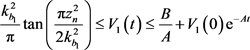(47)

$\frac{1}{2{\xi }_{1}}{\stackrel{˜}{\Theta }}_{1}^{2}\le {V}_{1}\left(t\right)\le \frac{B}{A}+{V}_{1}\left(0\right){\text{e}}^{-At}$ (48)

$|{z}_{1}|\le \sqrt{\frac{2{k}_{{b}_{1}}^{2}}{\text{π}}\mathrm{arctan}\left(\frac{\text{π}}{2{k}_{{b}_{1}}^{2}}\left(\frac{B}{A}+{V}_{1}\left(0\right){\text{e}}^{-At}\right)\right)}$ (49)

$|{\stackrel{˜}{\Theta }}_{1}|\le \sqrt{2{\xi }_{1}\left(\frac{B}{A}+{V}_{1}\left(0\right){\text{e}}^{-At}\right)}$ (50)

$\frac{{k}_{{b}_{2}}^{2}}{\text{π}}\mathrm{tan}\left(\frac{\text{π}{z}_{n}^{2}}{2{k}_{b2}^{2}}\right)\le {V}_{2}\left(t\right)\le \frac{B}{A}+{V}_{2}\left(0\right){\text{e}}^{-At}$ (51)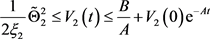(52)

$|{z}_{2}|\le \sqrt{\frac{2{k}_{{b}_{2}}^{2}}{\text{π}}\mathrm{arctan}\left(\frac{\text{π}}{2{k}_{{b}_{2}}^{2}}\left(\frac{B}{A}+{V}_{2}\left(0\right){\text{e}}^{-At}\right)\right)}$ (53)

3. 仿真实验

$\left\{\begin{array}{l}{\stackrel{˙}{x}}_{1}=0.4\mathrm{sin}\left({x}_{1}^{2}\right)+{x}_{2}\\ {\stackrel{˙}{x}}_{2}=0.1{\left({x}_{1}+{x}_{2}\right)}^{2}+u\left(v\right)\\ y={x}_{1}\end{array}$ (54)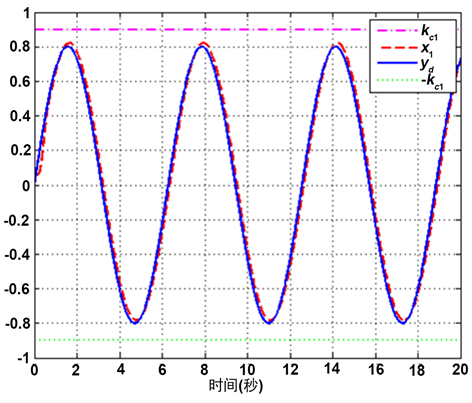Figure 1. The trajectories of the state x1 and the reference signal yd

$\begin{array}{l}{\alpha }_{1}=-\left(\frac{{\varphi }_{1}}{\text{π}{z}_{1}}{k}_{{b}_{1}}^{2}\right)\left(\mathrm{sin}\left(\frac{\text{π}{z}_{1}^{2}}{2{k}_{{b}_{1}}^{2}}\right)\mathrm{cos}\left(\frac{\text{π}{z}_{1}^{2}}{2{k}_{{b}_{1}}^{2}}\right)\right)-\frac{1}{2}{z}_{1}{\mathrm{sec}}^{2}\left(\frac{\text{π}{z}_{1}^{2}}{2{k}_{{b}_{1}}^{2}}\right)\\ \text{\hspace{0.17em}}\text{\hspace{0.17em}}\text{\hspace{0.17em}}\text{\hspace{0.17em}}\text{\hspace{0.17em}}\text{\hspace{0.17em}}\text{ }-\frac{1}{2}{z}_{1}{\mathrm{sec}}^{2}\left(\frac{\text{π}{z}_{1}^{2}}{2{k}_{{b}_{1}}^{2}}\right)-\frac{{\stackrel{^}{\Theta }}_{1}}{2{a}_{1}^{2}}{S}_{1}^{\text{T}}\left({Z}_{1}\right){S}_{1}\left({Z}_{1}\right){z}_{1}{\mathrm{sec}}^{2}\left(\frac{\text{π}{z}_{1}^{2}}{2{k}_{{b}_{1}}^{2}}\right)\\ v=\frac{{k}_{{b}_{2}}^{2}{\varphi }_{2}}{\text{π}{z}_{2}}\left(\mathrm{sin}\left(\frac{\text{π}{z}_{1}^{2}}{2{k}_{{b}_{1}}^{2}}\right)\mathrm{cos}\left(\frac{\text{π}{z}_{1}^{2}}{2{k}_{{b}_{1}}^{2}}\right)\right)+\frac{{\stackrel{^}{\Theta }}_{2}}{2{a}_{2}^{2}}{S}_{2}^{\text{T}}\left({Z}_{2}\right){S}_{2}\left({Z}_{2}\right){z}_{2}{\mathrm{sec}}^{2}\left(\frac{\text{π}{z}_{2}^{2}}{2{k}_{{b}_{2}}^{2}}\right)\\ \text{\hspace{0.17em}}\text{\hspace{0.17em}}\text{\hspace{0.17em}}\text{\hspace{0.17em}}\text{\hspace{0.17em}}+\frac{1}{2}{z}_{2}{\mathrm{sec}}^{2}\left(\frac{\text{π}{z}_{2}^{2}}{2{k}_{{b}_{2}}^{2}}\right)+\frac{1}{2}{\mathrm{sec}}^{2}\left(\frac{\text{π}{z}_{2}^{2}}{2{k}_{{b}_{2}}^{2}}\right)+\frac{1}{2}{z}_{2}{\mathrm{cos}}^{2}\left(\frac{\text{π}{z}_{2}^{2}}{2{k}_{{b}_{2}}^{2}}\right)\right)\end{array}$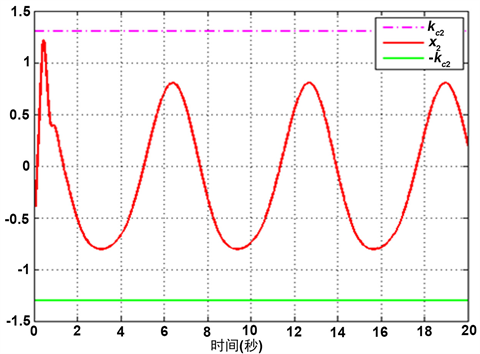Figure 2. The trajectory of the state x2

${\stackrel{˙}{\stackrel{^}{\Theta }}}_{j}=\frac{{\xi }_{j}}{2{a}_{j}^{2}}{S}_{j}^{\text{T}}\left({Z}_{j}\right){S}_{j}\left({Z}_{j}\right){z}_{j}^{2}{\mathrm{sec}}^{4}\left(\frac{\text{π}{z}_{j}^{2}}{2{k}_{{b}_{j}}^{2}}\right)-{\sigma }_{j}{\stackrel{^}{\Theta }}_{j},j=1,2$Figure 3. The phase trajectories of the errors z1 and z2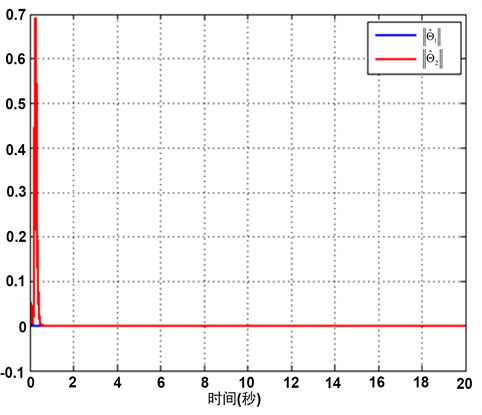Figure 4. The curves of the adaptive laws ${\stackrel{^}{\Theta }}_{1}$ and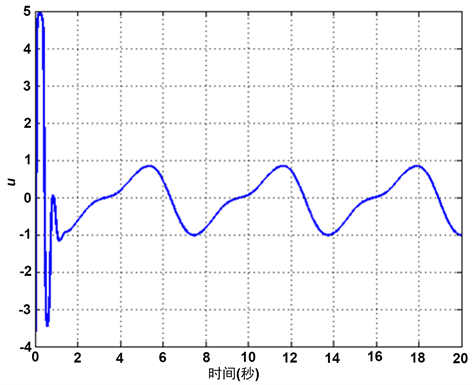Figure 5. The curve of the system input u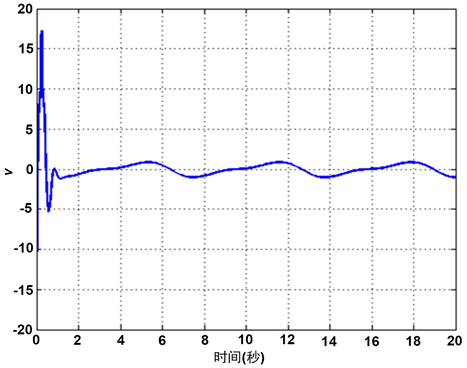Figure 6. The trajectory of the saturated input v

4. 结论

Constrained Control for Strict Feedback Systems with Input Saturation[J]. 建模与仿真, 2019, 08(03): 102-116. https://doi.org/10.12677/MOS.2019.83013

1. 1. 武晓晶. 具有输入输出约束特性的非线性系统自适应控制研究[D]: [博士学位论文]. 秦皇岛: 燕山大学, 2012.

2. 2. 王敏. 具有时变输出约束的非线性系统鲁棒自适应控制研究[D]: [硕士学位论文]. 扬州: 扬州大学, 2018.

3. 3. 唐忠樑. 具有输出约束和状态约束的不确定非线性系统的自适应神经网络控制及其应用[D]: [博士学位论文]. 成都: 电子科技大学, 2016.

4. 4. Wang, C. and Wu, Y. (2019) Finite-Time Tracking Control for Strict-Feedback Nonlinear Systems With Full State Constraints. International Journal of Control, 92, 1426-1433. https://doi.org/10.1080/00207179.2017.1397290

5. 5. Tee, K.P., Ge, S.S. and Tay, E.H. (2009) Barrier Lyapunov Functions for the Control of Output-Constrained Nonlinear Systems. Automatica, 45, 918-927. https://doi.org/10.1016/j.automatica.2008.11.017

6. 6. Jin, X. (2016) Adaptive Fault Tolerant Control for a Class of Input and State Constrained MIMO Nonlinear Systems. International Journal of Robust & Nonlinear Control, 26, 286-302. https://doi.org/10.1002/rnc.3312